# pareto

•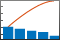## Syntax

``pareto(y)``
``pareto(y,x)``
``pareto(___,threshold)``
``pareto(target,___)``
``charts = pareto(___)``
``[charts,ax] = pareto(___)``

## Description

example

````pareto(y)` creates a Pareto chart of `y`. A Pareto chart is a bar chart with the bars sorted in descending order, and it includes a line that shows the cumulative distribution. The chart displays the tallest bars that comprise 95% of the cumulative distribution, up to a maximum of `10` bars. If `n` bars contain exactly 95% of the distribution, and `n` is less than `10`, the chart displays `n+1` bars.The bar labels along the x-axis are the indices to the bar values in the `y` vector.```

example

````pareto(y,x)` specifies the x-coordinates (or labels) for the bars. Both `y` and `x` must be the same length.```

example

````pareto(___,threshold)` specifies a threshold value between `0` and `1`. The threshold is the fraction of the cumulative distribution to include in the chart. The chart includes the tallest bars that comprise that fraction, up to a maximum of `10` bars. If `n` bars contain exactly the specified fraction, and `n` is less than `10`, the chart displays `n+1` bars.```

example

````pareto(target,___)` creates the chart in the specified target axes.```
````charts = pareto(___)` returns the `Bar` and `Line` objects in an array. You can use the objects to modify aspects of the Pareto chart by setting properties. For a list of properties, see Bar Properties and Line Properties.```

example

````[charts,ax] = pareto(___)` returns an array of two `Axes` objects in addition to the objects in the `charts` array. You can use these objects to modify aspects of the chart by setting properties. For a list of properties, see Axes Properties, Bar Properties, and Line Properties.```

## Examples

collapse all

Define `y` as a vector of five numbers that sum to 100, so you can see the relationship between the numbers and the bars in the chart.

Then, create a Pareto chart of `y` without specifying the x-coordinates. The x-axis tick labels are the locations of the bar values in `y`. In this case, `y(3)` is the largest value, so its bar displays in the left most position, followed by `y(5)` and `y(4)`. These three bars comprise more than 95% of the cumulative distribution, so they are the only bars shown in the chart.

```y = [2 3 45 20 32]; pareto(y)```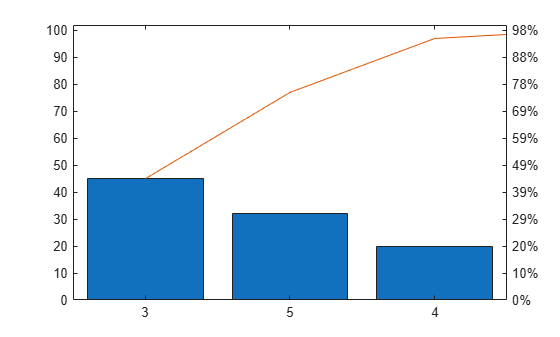When `n` of the tallest bars comprise exactly 95% of the cumulative distribution, `pareto` includes `n+1` bars in the chart. For example, define `y` such that two bars contain exactly 95% of the data. `pareto` displays the tallest three bars.

```y = [4 1 40 55]; pareto(y)```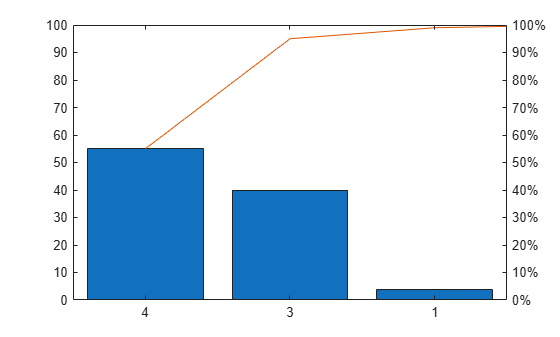Use a Pareto chart to examine the preferred types of pie in a survey of 200 participants. Define `x` as a string vector containing five pie flavors, and define `y` as the number of votes for each flavor. Create a Pareto chart, and include all the values in the cumulative distribution by setting the `threshold` argument to `1`.

```x = ["Chocolate" "Apple" "Pecan" "Cherry" "Pumpkin"]; y = [35 50 30 5 80]; pareto(y,x,1) ylabel('Votes')```Define `x` as a string vector containing the names of eight programmers that contributed to a project. Define `y` as the number of lines of code that each programmer contributed. Display the data in a Pareto chart with a title.

```x = ["Fred" "Gina" "Norman" "Josphat" "Julia" "Wally" "Heidi" "Pat"]; y = [200 120 555 608 1024 101 57 687]; pareto(y,x) title('Lines of Code by Programmer')```Define `x` as a categorical vector of the names of five different model rockets manufactured at a particular factory. Define `y` as the number of rockets that failed to launch from a random sampling within each category. Display the data in a Pareto chart, and add labels to the x- and y-axes.

```x = categorical(["Firestorm" "Mr. Ballista" "Moonshot" "Lil' Joe" "Houston"]); y = [526 100 221 40 10]; pareto(y,x) xlabel('Rocket Model') ylabel('Launch Failures')```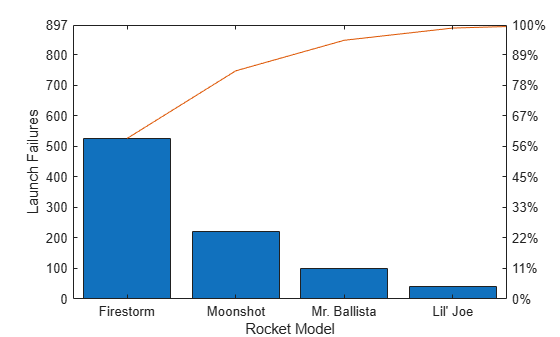Define `x` as a datetime vector indicating the manufacturing dates for a particular electronic component. Define `y` as the number of defects for each batch of components. Display the data in a Pareto chart, and add labels to the x- and y-axes.

```x = datetime(2018,5,1:5,'Format','d MMM'); y = [100 526 221 60 49]; pareto(y,x) xlabel('Manufacturing Date') ylabel('Defects')```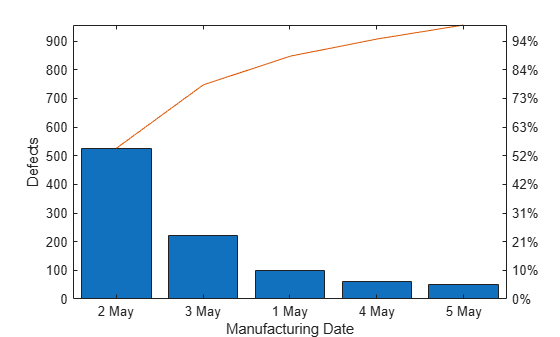Define `y` as a vector of five numbers, and create a Pareto chart. Specify return arguments so you can customize aspects of the chart and axes.

```y = [20 30 10 55 5]; [charts, ax] = pareto(y);```Use the `charts` array to change the colors of the `Bar` and the `Line` objects. The first element in `charts` is the `Bar` object, and the second element is the `Line` object. Change the bar colors to a shade of purple, and change the line color to a shade of green.

```charts(1).FaceColor = [0.50 0.37 0.60]; charts(2).Color = [0 0.50 0.10];```Next, change the color of the left y-axis to match the bars, and change the color of the right y-axis to match the line. The `Axes` object for the left side is the first element of the `ax` array. The other `Axes` object is in the second element of the `ax` array.

After changing the colors, display the axes grid lines.

```ax(1).YColor = [0.50 0.37 0.60]; ax(2).YColor = [0 0.50 0.10]; grid on```To create multiple charts in a figure, use a tiled chart layout. Call the `nexttile` function to create an axes object in a tiled chart layout. If there is no layout available, `nexttile` creates one. Create a Pareto chart by passing the axes to the `pareto` function as the first argument.

```ax1 = nexttile; pareto(ax1,[20 50 33 12])```Call the `nexttile` function to add a second axes object to the layout. Then create a second Pareto chart.

```ax2 = nexttile; pareto(ax2,[50 10 20 25 30])```## Input Arguments

collapse all

y-coordinates, or bar heights, specified as a vector of finite, nonnegative, numeric values.

Data Types: `single` | `double` | `int8` | `int16` | `int32` | `int64` | `uint8` | `uint16` | `uint32` | `uint64`

x-coordinates, or bar labels, specified as a vector the same length as `y`. The values in the vector can be of finite, nonnegative, numeric values, datetime values, duration values, or categorical values.

Data Types: `single` | `double` | `int8` | `int16` | `int32` | `int64` | `uint8` | `uint16` | `uint32` | `uint64` | `categorical` | `datetime` | `duration`

Fraction of the cumulative distribution to include in the chart, specified as a number between `0` and `1`.

`pareto` displays the bars that contribute to the cumulative distribution in descending order, until just beyond the threshold, up to a maximum of `10` bars.

Example: `pareto([70 15 10 5],0.75)` displays the tallest bars that contain 75% of the cumulative distribution.

Example: `pareto([70 15 10 5],0.85)` displays the tallest bars that contain 85% of the cumulative distribution.

Example: `pareto([70 15 10 5],1)` displays all the bars, since the length of `y` is less than 10.

Example: `pareto(1:11,1)` displays only the tallest 10 bars, since the length of `y` is greater than 10.

Target axes, specified as an `Axes` object. If you do not specify the axes, and if the current axes is Cartesian, `pareto` uses the current axes.

## Version History

Introduced before R2006a